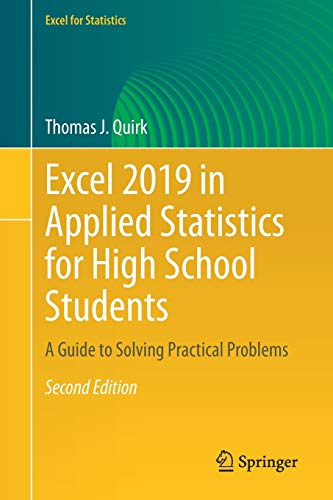# Excel 2019 in Applied Statistics for High School Students, 2nd Edition

Rating: 0 out of 5 0 reviews
• Length: 264 pages
• Edition: 2
• Publisher:
• Publication Date: 2021-03-16
• ISBN-10: 3030667553
• ISBN-13: 9783030667559

## Book Description

This textbook is a step-by-step guide for high school, community college, and undergraduate students who are taking a course in applied statistics and wish to learn how to use Excel to solve statistical problems. All of the statistics problems in this book come from the following fields of study: business, education, psychology, marketing, engineering and advertising. Students will learn how to perform key statistical tests in Excel without being overwhelmed by statistical theory.

Each chapter briefly explains a topic and then demonstrates how to use Excel commands and formulas to solve specific statistics problems. The book offers guidance in using Excel in two different ways: (1) writing formulas (e.g., confidence interval about the mean, one-group t-test, two-group t-test, correlation) and (2) using Excel’s drop-down formula menus (e.g., simple linear regression, multiple correlations and multiple regression, and one-way ANOVA). Three practice problems are provided at the end of each chapter, along with their solutions in an appendix. An additional practice test allows readers to test their understanding of each chapter by attempting to solve a specific statistics problem using Excel; the solution to each of these problems is also given in an appendix. This book is a tool that can be used either by itself or along with any good statistics book.​

``````1. Sample Size, Mean, Standard Deviation, and Standard Error of the Mean